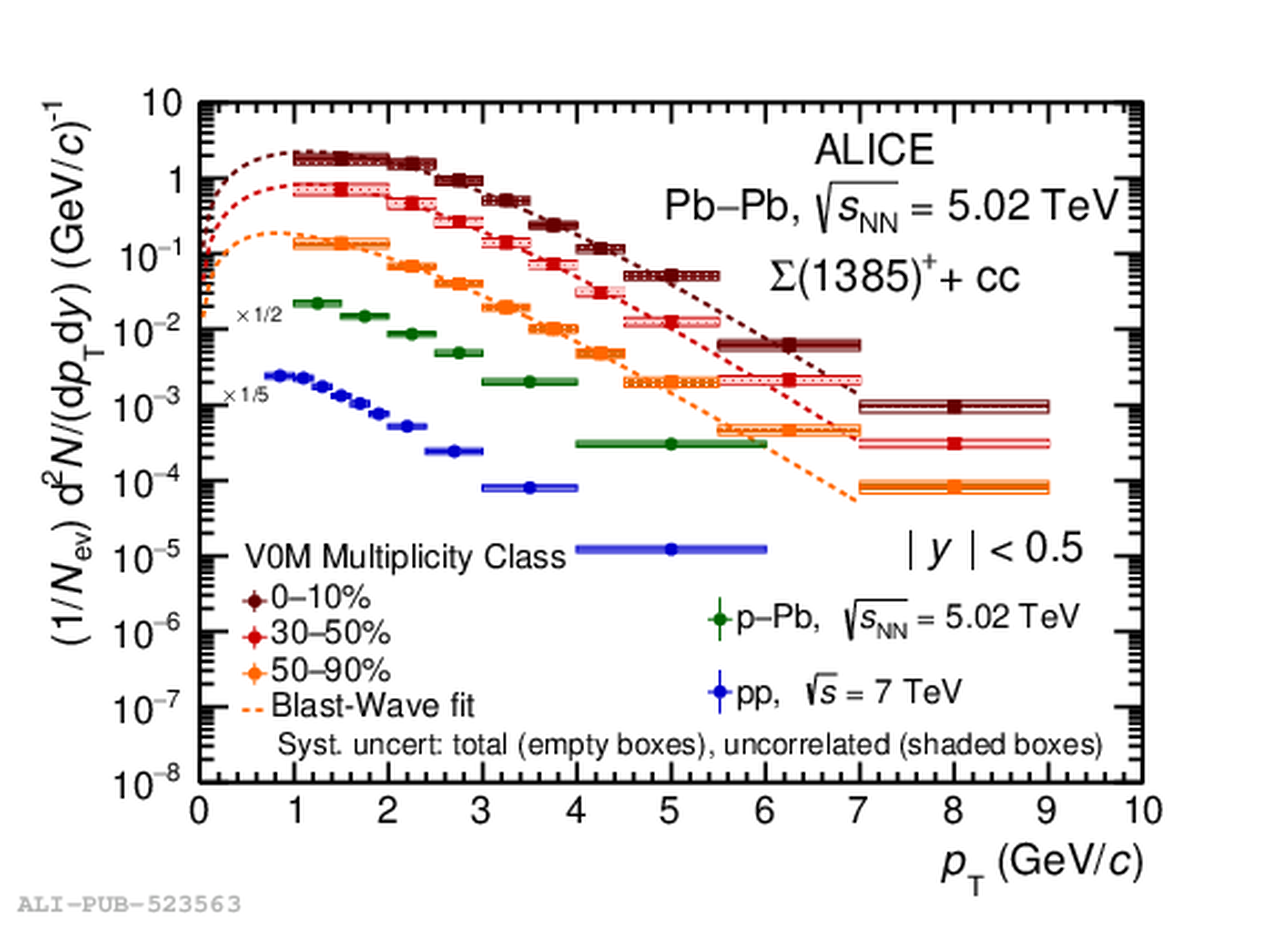# Figure 3

 $p_{\rm T}$ spectra of $\Sigma(1385)^{+}$ (left) and $\Sigma(1385)^{-}$ (right) resonances in \PbPb collisions at \fivenn in three centrality classes. Empty and shaded boxes depict the total and uncorrelated uncertainties, respectively. The Blast-Wave fit functions are plotted up to 7 \GeVc. The measurements in \pp collisions at \seven  and in \pPb collisions at \five (NSD)  are quoted for comparisons.